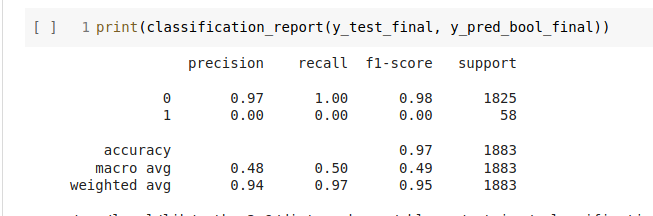# Why precision/recall worsens while validation loss continue to improve?

I am trainig a CNN model for binary class, with class imbalance. On trainig, accuracy imporves dramatically. But on deeper inspection of precision and recall, the model performs terrible, as is it doesnt learn class two at all(which is expected due to imbalance)

Using -Xception model , Image BInary classification , (model frozen partially)

Prediction layer used

predictions = Dense(1, activation = “sigmoid”)

Data also shows Class imbalance, one call being 15000 , another being 500 images.

Used image augmentation too.

``````datagen = ImageDataGenerator(rescale=1.0/255.0, rotation_range=50, width_shift_range=0.3,
zoom_range=0.2,horizontal_flip=True, vertical_flip=True,fill_mode='nearest',
validation_split= validation_split, )
train_gen = datagen.flow_from_dataframe(df, directory= directory,
x_col='image_name', y_col='label' , class_mode='raw' ,shuffle= True , subset='training')
val_gen = datagen.flow_from_dataframe(df, directory= directory,
x_col='image_name', y_col='label'     , class_mode='raw' , shuffle= True ,subset='validation')
``````
``````def recall(y_true, y_pred):
y_true = K.ones_like(y_true)
true_positives = K.sum(K.round(K.clip(y_true * y_pred, 0, 1)))
all_positives = K.sum(K.round(K.clip(y_true, 0, 1)))
recall = true_positives / (all_positives + K.epsilon())
return recall

def precision(y_true, y_pred):
y_true = K.ones_like(y_true)
true_positives = K.sum(K.round(K.clip(y_true * y_pred, 0, 1)))

predicted_positives = K.sum(K.round(K.clip(y_pred, 0, 1)))
precision = true_positives / (predicted_positives + K.epsilon())
return precision

def f1_score(y_true, y_pred):
precision1 = precision(y_true, y_pred)
recall1 = recall(y_true, y_pred)
return 2*((precision1*recall1)/(precision1+recall1+K.epsilon()))
``````
``````Adamoptimizer = tf.keras.optimizers.Adam(learning_rate=learning_rate, beta_1=0.9, beta_2=0.999, amsgrad=False)

History = model.fit(train_gen, validation_data=val_gen, steps_per_epoch= 10,
validation_steps= 5, epochs=epoch , shuffle =True)

metrics=[ recall ,precision] )
``````

Earlier i used accuracy as a metrics, but it showed me 98, 99+ accuracy and val_accuracy , but performed poorely on test dataset. Attached as an imageHere is my training results.
(P.S. - I have trained on lesser steps per epoch , to observe the trend of Recall and precision)
Epoch 1/20
10/10 [==============================] - 14s 1s/step - loss: 0.6895 - recall: 0.4750 - precision: 1.0000 - val_loss: 0.7395 - val_recall: 0.6219 - val_precision: 1.0000
Epoch 2/20
10/10 [==============================] - 12s 1s/step - loss: 0.6590 - recall: 0.2812 - precision: 1.0000 - val_loss: 0.6704 - val_recall: 0.3844 - val_precision: 1.0000
Epoch 3/20
10/10 [==============================] - 12s 1s/step - loss: 0.6212 - recall: 0.1094 - precision: 1.0000 - val_loss: 0.6180 - val_recall: 0.1625 - val_precision: 1.0000
Epoch 4/20
10/10 [==============================] - 12s 1s/step - loss: 0.5801 - recall: 0.0250 - precision: 0.7000 - val_loss: 0.5768 - val_recall: 0.0719 - val_precision: 0.9000
Epoch 5/20
10/10 [==============================] - 12s 1s/step - loss: 0.5391 - recall: 0.0063 - precision: 0.2000 - val_loss: 0.5335 - val_recall: 0.0281 - val_precision: 0.4000
Epoch 6/20
10/10 [==============================] - 12s 1s/step - loss: 0.4943 - recall: 0.0000e+00 - precision: 0.0000e+00 - val_loss: 0.4831 - val_recall: 0.0094 - val_precision: 0.3000
Epoch 7/20
10/10 [==============================] - 12s 1s/step - loss: 0.4476 - recall: 0.0000e+00 - precision: 0.0000e+00 - val_loss: 0.4607 - val_recall: 0.0031 - val_precision: 0.1000
Epoch 8/20
10/10 [==============================] - 12s 1s/step - loss: 0.4019 - recall: 0.0000e+00 - precision: 0.0000e+00 - val_loss: 0.4208 - val_recall: 0.0000e+00 - val_precision: 0.0000e+00
Epoch 9/20
10/10 [==============================] - 12s 1s/step - loss: 0.3508 - recall: 0.0000e+00 - precision: 0.0000e+00 - val_loss: 0.3754 - val_recall: 0.0000e+00 - val_precision: 0.0000e+00
Epoch 10/20
10/10 [==============================] - 12s 1s/step - loss: 0.3065 - recall: 0.0000e+00 - precision: 0.0000e+00 - val_loss: 0.3459 - val_recall: 0.0000e+00 - val_precision: 0.0000e+00
Ep

I read these blogs, they are realted, but didnt help.What can be probable cause of reduction of recall and precision? And how can i fix it??

Also, what are ways to deal with class imbalance.
Have you tried using `weight` in the loss function? https://discuss.pytorch.org/t/per-class-and-per-sample-weighting/25530/2. You could use the reference from https://scikit-learn.org/stable/modules/generated/sklearn.utils.class_weight.compute_class_weight.html to figure out how to weight it, or just do a ratio of the positive to negative (15000 : 500)